# Cone roof

How many m2 of roofing is needed to cover a cone-shaped roof with a diameter of 10 m and a height of 4 m? Add an extra 4% to the overlays.

Result

S =  104.603 m2

#### Solution:

$D=10 \ \text{m} \ \\ h=4 \ \text{m} \ \\ q=4 \%=1 + \dfrac{ 4 }{ 100 }=1.04 \ \\ \ \\ r=D/2=10/2=5 \ \text{m} \ \\ s=\sqrt{ h^2+r^2 }=\sqrt{ 4^2+5^2 } \doteq \sqrt{ 41 } \ \text{m} \doteq 6.4031 \ \text{m} \ \\ \ \\ S_{1}=\pi \cdot \ r \cdot \ s=3.1416 \cdot \ 5 \cdot \ 6.4031 \doteq 100.58 \ \text{m}^2 \ \\ \ \\ S=q \cdot \ S_{1}=1.04 \cdot \ 100.58 \doteq 104.6032 \doteq 104.603 \ \text{m}^2$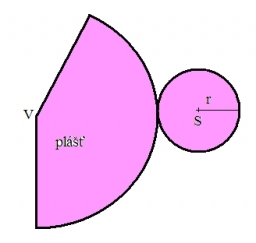Our examples were largely sent or created by pupils and students themselves. Therefore, we would be pleased if you could send us any errors you found, spelling mistakes, or rephasing the example. Thank you!

Leave us a comment of this math problem and its solution (i.e. if it is still somewhat unclear...):Be the first to comment!Tips to related online calculators
Pythagorean theorem is the base for the right triangle calculator.

#### You need to know the following knowledge to solve this word math problem:

We encourage you to watch this tutorial video on this math problem:

## Next similar math problems:

1. Storm and roof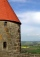The roof on the building is a cone with a height of 3 meters and a radius equal to half the height of the roof. How many m2 of roof need to be repaired if 20% were damaged in a storm?
2. Roof of the church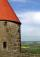The cone roof of the church has a diameter of 3m and a height of 4m. What is the size of the side edge of the church roof (s) and how much sheet will be needed to cover the church roof?
3. Cone 15The radius of the base of a right circular cone is 14 inches and it's height 18 inches. What is the slant height?
4. The bus stop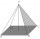The bus stop waiting room has the shape of a regular quadrilateral pyramid 4 m high with a 5 m base edge. Calculate how many m2 roofing is required to cover the three walls of the sheathing, taking into account 40% of the additional coverage.
5. Church roof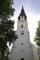The roof of the church tower has the shape of a regular tetrahedral pyramid with base edge length 5.4 meters and a height 5 m. It was found that needs to be corrected 27% covering of the roof area. What amount of material will be required?
6. SummerjobThe temporary workers planted new trees. Of the total number of 500 seedlings, they managed to plant 426. How many percents did they meet the daily planting limit?
7. Researchers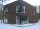Researchers ask 200 families whether or not they were the homeowner and how many cars they had. Their response was homeowner: 14 no car or one car, two or more cars 86, not homeowner: 38 no car or one car, two or more cars 62. What percent of the families
8. Triangle IRTIn isosceles right triangle ABC with right angle at vertex C is coordinates: A (-1, 2); C (-5, -2) Calculate the length of segment AB.
9. Triangle ABCIn a triangle ABC with the side BC of length 2 cm The middle point of AB. Points L and M split AC side into three equal lines. KLM is isosceles triangle with a right angle at the point K. Determine the lengths of the sides AB, AC triangle ABC.
10. Euclid2In right triangle ABC with right angle at C is given side a=27 and height v=12. Calculate the perimeter of the triangle.
11. Profit gainIf 5% more is gained by selling an article for Rs. 350 than by selling it for Rs. 340, the cost of the article is:
12. Right 24Right isosceles triangle has an altitude x drawn from the right angle to the hypotenuse dividing it into 2 unequal segments. The length of one segment is 5 cm. What is the area of the triangle? Thank you.
13. Euclid 5Calculate the length of remain sides of a right triangle ABC if a = 7 cm and height vc = 5 cm.
14. RT triangle and heightCalculate the remaining sides of the right triangle if we know side b = 4 cm long and height to side c h = 2.4 cm.The double ladder is 8.5m long. It is built so that its lower ends are 3.5 meters apart. How high does the upper end of the ladder reach?Calculate the absolute value of complex number -15-29i.How tall was spruce that was cut at an altitude of 8m above the ground and the top landed at a distance of 15m from the heel of the tree?